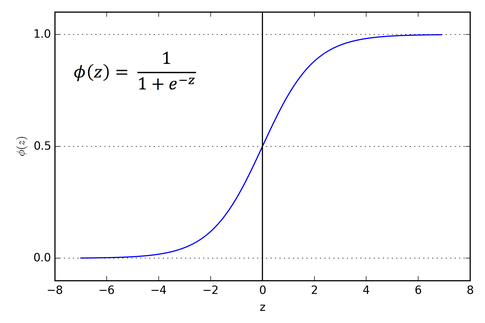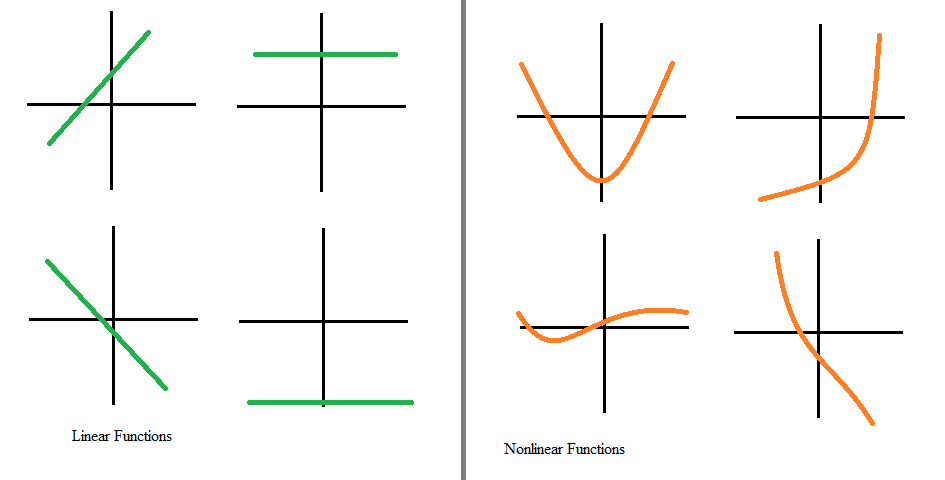# Sigmoidal Nonlinearity

## Sigmoidal Nonlinearity

The name Sigmoidal refers to the Greek letter Sigma, and when graphed, resembles a sloping “S” across the Y-axis. A sigmoidal function is a type of logistic function and purely refers to any function that retains the “S” shape, such as the hyperbolic tangent function,  tanh(x).  The main utility of this class of functions is that they are smooth versions of a step function, meaning that the derivative exists everywhere.  This is important for neural networks as learning is typically requires computing the gradients (partial derivatives) through the backpropagation technique.  Where a traditional sigmoidal function exists between 0 and 1, tanh(x) follows a similar shape, but exists between 1 and -1,  which can have computational advantages.(Fig: Sigmoid Function)

Nonlinearity means the output is not simply a constant scaling of the input variables (constant slope), i.e. the rate of change is not proportional across all independent variable.  Here are some examples of linear vs. nonlinear functions:(image is taken from Study.com)

## How Does Machine Learning Apply Sigmoidal Nonlinearity?

The use of sigmoidal nonlinear functions was inspired by the ouputs of biological neurons.  In the brain, the output of a neuron is typically all or nothing (on or off), and hence, can mathematically be modeled as a function with only two outputs.  Since neurons begin to fire (turn on) after a certain input threshold has been surpassed, the simplest mathematical function to model this behavior is the (Heaviside) step function, which outputs zero below a threshold input value and outputs one above the threshold input value.  However, this function is not smooth (it fails to be differential at the threshold value).  Therefore, the sigmoid class of functions is a differentiable alternative that still captures much of the behavior of biological neurons.

Sigmoidal functions are known generally as activation functions, and more specifically as a squashing functions. The "squashing" refers to the fact that the output of the function exists between a finite limit, usually 0 and 1. These functions are incredibly useful in determining probability

Sigmoidal functions are frequently used in machine learning, specifically to model the output of a node or “neuron.”   These functions are inherently non-linear and thus allow neural networks to find non-linear relationships between data features.  This greatly expands the utility of neural networks and allows them (in principle) to learn any function.